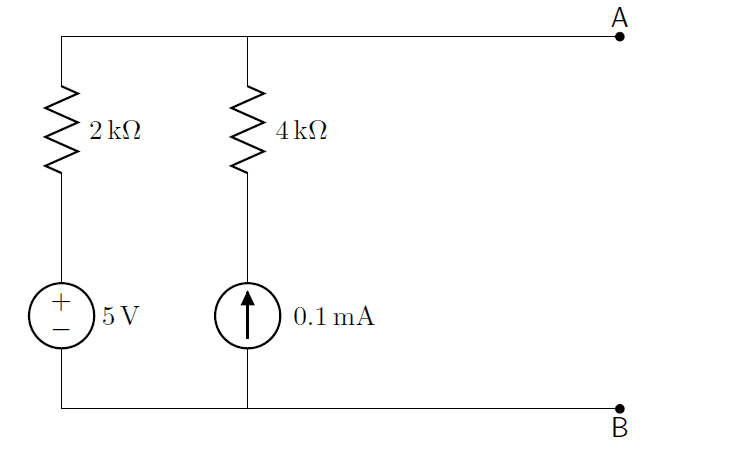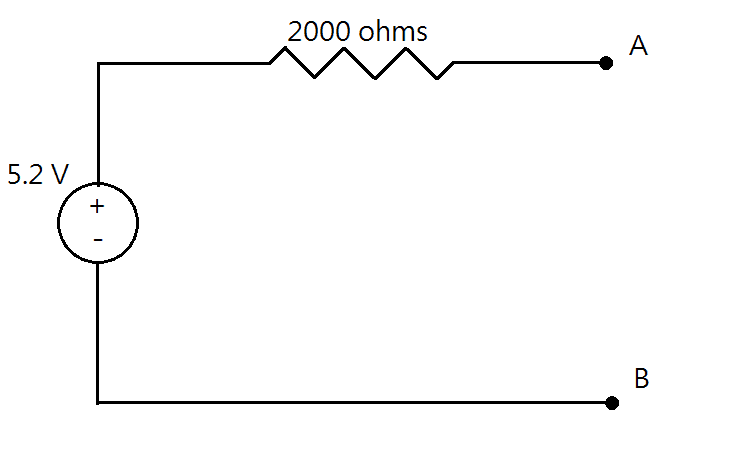# Thevenin's and Norton's Theorem

Hi. It's me again. Right now I have some troubles with Thevenin's and Norton's theorem.
Here is the circuit.I have to find the thevenin voltage Vth and thevenin resistance Rth and solve the circuit when there is a 2000Ω resistor connected to A and B.
Then, I try to solve it...

First, for the Rth, I just treat the voltage source as a short circuit and current source as an open circuit. So, the 4000Ω resistor is disconnected.

Rth = 2000Ω

Then, for the Vth, Vth = Voc.
By using KCL,

5/2000 + 0.1×10-3 = Voc/Rth

5/2000 + 0.1×10-3 = Voc/2000

∴ Voc = 5.2 V
i.e. Vth = 5.2 VIs this correct?

Last edited:

NascentOxygen
Staff Emeritus
Rth = 2000Ω

Then, for the Vth, Vth = Voc.
By using KCL,

5/2000 + 0.1×10-3 = Voc/Rth

5/2000 + 0.1×10-3 = Voc/2000

∴ Voc = 5.2 V
i.e. Vth = 5.2 V
Looks right.So what will the Norton equivalent be?

psparky
Gold Member
Is this correct?

You could also use superposition as well. The voltage source is straight forward at 5 volts (open current source)

The current source is a bit more tricky( short voltage source). .1 amp thru 4K resistor is .4 volts....but you then put it thru the 2K resistor and its .2 volts in th opposite direction.

How can you have two different voltages in two paralell branches?

Must mean there is .2 volts across the current source in the opposite direction of the 4K resistor.
5 +.2 = 5.2 Volts. Or you could do the way above, but superposition is just another way to enjoy and undestand the problem.

IN = 2.6 x 10-3 A and RN = Rth